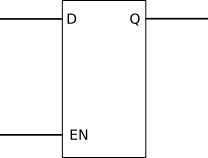It is that time of the year when we need to pay for web hosting and buy new components and equipment for new tutorials. You can help by making a donation. Contribute to this website by clicking the Donate button. The total will be updated once daily. (You may need to clear your browser cache to see the updates.)Target Amount: \$2000
Amount Raised: \$1802.10

Top Donor: C.C. \$100

X

# Tutorial 10: Gated D Latch in VHDL

Created on: 22 January 2013

A gated D type latch is written in VHDL code and implemented on a CPLD. Two different ways are used to implement the same latch.

## Gated D Latch Operation

The logic symbol for a gated D latch is shown below.Gated D Latch Logic Symbol

On the above gated D latch, D is the data input, Q is the data output and EN is the active high enable.

The gated D latch will only reflect the D input on the Q output when EN is active. When EN is not active, the last state of Q is latched (the last state of Q when EN was last active).

This video shows the gated D latch in operation:

Can't see the video? View on YouTube →

## Gated D Latch VHDL Code

There is usually more than one way to implement a logic design in VHDL code. The same D latch is implemented in two different ways below to illustrate this point.

### First D Latch

The VHDL code listing for the first VHDL D latch is shown here.

```library IEEE;
use IEEE.STD_LOGIC_1164.ALL;

entity d_latch_top is
Port ( D  : in  STD_LOGIC;
EN : in  STD_LOGIC;
Q  : out STD_LOGIC);
end d_latch_top;

architecture Behavioral of d_latch_top is
signal DATA : STD_LOGIC;
begin

DATA <= D when (EN = '1') else DATA;
Q <= DATA;

end Behavioral;
```

In the above VHDL code, the signal DATA is used to store the state of the data that is to be latched. DATA is made to be the same as the D input when EN is high. When EN is low, DATA will equal DATA – in other words the value that was last stored in data when EN was high is now latched in DATA.

The Q output of the latch is made to reflect the logic state stored in DATA.

### Second D Latch

The VHDL code listing for the second VHDL D latch is shown here.

```library IEEE;
use IEEE.STD_LOGIC_1164.ALL;

entity d_latch_2_top is
Port ( EN : in  STD_LOGIC;
D  : in  STD_LOGIC;
Q  : out STD_LOGIC);
end d_latch_2_top;

architecture Behavioral of d_latch_2_top is
begin

process (EN, D)
begin
if (EN = '1') then
Q <= D;
end if;
end process;

end Behavioral;
```

This second VHDL code gated D latch is implemented in a VHDL process. In the process, if EN is high, then the D input is reflected on the Q output. When EN is low nothing happens, which means that Q stays at the same logic level it was at when EN was high – no matter whether the logic level on the D input changes.

## A Word About Latches in VHDL

It is not considered good practice to implement or infer latches in VHDL code. In fact the Xilinx tools give a warning about latches when the above two VHDL code listings are compiled. The D latches and the S-R latch from the previous tutorial have only been used for learning about VHDL code.

When doing logic designs in VHDL, it is best to use registers instead of latches. We will look at registers in more detail later in this course.

Amazon.com

Amazon.co.uk